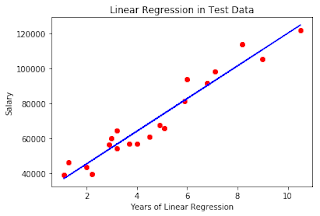Using Mathematics to find the price of a house - That Computer Engineer

Friday, April 27, 2018

Statistics and Computer skills can do wonder to you and make your life easier. One such application is to predict the housing price for the area. Suppose you are looking for a house. So there will be a lot of factors that will affect the price of the house in a certain area. Various such features include:

• Location
• Rooms available
• Parking
• Neighborhood
• Size
• Age of house
• Closeness to market and other necessary areas
Before introducing the concept of deciding the price of a house, let me introduce you to the topic of Linear regression and multiple regression, which is, in fact, the basic concept behind deciding the price of a house. Although its one of the many ways of deciding and not the only way.

Linear Regression

This is a simple concept. There are only two variables involved in linear regression -• Independent variable: Here the independent variable will be the basic deciding factors that will affect the price of a house.
Example: Size, Number of rooms, Parking available or not, etc
• Dependent variable: This is the output that we want to conclude based on the dependent variables. The independent variable depends on one or more dependent variable.
Example: Price of the house

y=b+b1x1

Here,
y is the dependent variable
x1 is the independent variable
b0 and b1 are the constants.

Consider a given example, where we are able to predict the salary of a person based on the years of experience for the person.

You see his at Blue line it is the prediction we made for the Predicted Salary of the person, based on the Years of experience. The red dots represent the Real Salary.

Similarly, by using the number of rooms in the house as the dependent variable (x-axis) and the price of the house as the independent variable (y-axis), we can represent a similar representation.

Multiple Regression

In multiple regression, instead of only one dependent variable and one independent variable, there is more than one independent variable that can put their effect on the conclusion of a dependent variable.

Example:

For predicting the price of a house,

Dependent variable: Price of a house
Independent variable: Size, Number of rooms, Neighbourhood, Parking presence (yes or no)

1 comment:

1.Nice article.